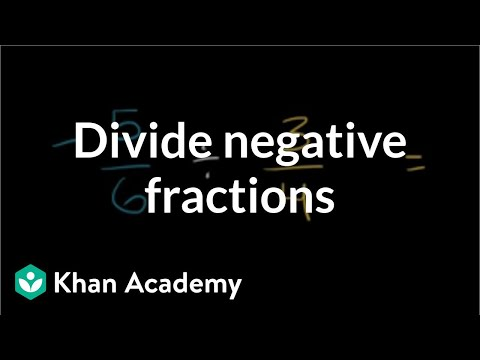Video

# Dividing positive and negative numbers (Full video)

Khan Academy

## Supporting grades: 7

Description: Discover the basics of dividing with negative numbers. Created by Sal Khan. Now that we know a little bit about multiplying positive and negative numbers, Let's think about how how we can divide them. And if both are negative, they'll cancel out and you'll get a positive answer. So if I just said eight (8) divided by two (2), that would be a positive four (4), but since exactly one of these two numbers are negative, this one right over here, the answer is going to be negative.

You must log inorsign upif you want to:*

*Teacher Advisor is 100% free.

### Other videos you might be interested in### Dividing negative fractions (Full video)

#### Khan Academy